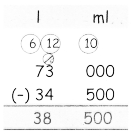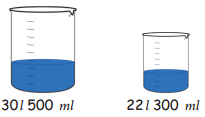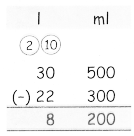Tamilnadu State Board New Syllabus Samacheer Kalvi 4th Maths Guide Pdf Term 3 Chapter 3 Measurements Ex 3.3 Textbook Questions and Answers, Notes.

## Tamilnadu Samacheer Kalvi 4th Maths Solutions Term 3 Chapter 3 Measurements Ex 3.3

Question 1.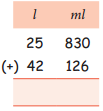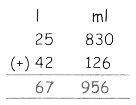Question 2.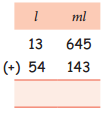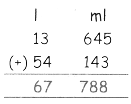Question 3.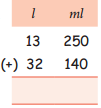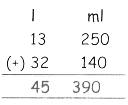II. Subtract the following
Question 1.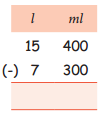Question 2.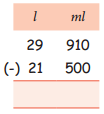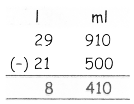Question 3.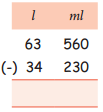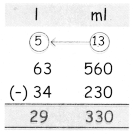III Solve the following.
Question 1.
Ramu filled the petrol in his two cars. The first one hold the capacity of 23 l 500 ml and the second one hold the capacity 15 l 750 ml. Find the total quantity of petrol.
First Car = 23 l 500 ml
Second Car = 15 l 750 ml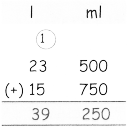Total Quantity = 39 l 250 mlQuestion 2.
Kannan has some cows. They give milk in the first week 48 1 480 ml and in the second week 57 1 530 ml. Find the total capacity of milk.
First week = 48 l 480 ml
Second week = 57 l 530 ml
Total = 106 l 10 ml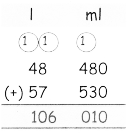Question 3.
The fruit juice used in a function are given below.

 S.No Cool drinks Capacity 1. Apple Juice 16 l 500 ml 2. Mango juice 23 l 160 ml 3. Grapes juice 19 l 650 ml 4. Lemon Juice 20 l 350 ml

1. Apple juice + Lemon juice = ———– l ———– ml
2. Mango juice + Grapes juice = ———– l ———– ml
3. Lemon juice + Mango juice = ———– l ———– ml
1. Apple juice + Lemon juice = ———– l ———– ml
Apple juice = 16 l 500 ml
Lemon juice = 20 l 350 ml
Total = 36 l 850 ml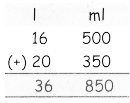2. Mango juice + Grapes juice = ———– l ———– ml
Mango juice = 23 l 160 ml
Grapes juice = 19 l 650 ml
Total = 42 l 810 ml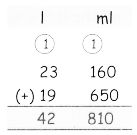3. Lemon juice + Mango juice = ———– l ———– ml
Lemon juice = 20 l 350 ml
Mango juice = 23 l 160 ml
Total = 43 l 510 ml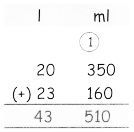Question 4.
The shop keeper had 43 l 750 ml of groundnut oil. He sold 24 l 350 ml. How much oil was left with him?
shop keeper had = 43 l 750 ml
sold = 24 l 350 ml
Left = 19 l 400 ml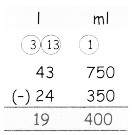Question 5.
A bucket contains 15 l 500 ml of water. Gopi poured out 5 l 200 m to water the plants. How much water is left in the bucket?
Bucket contains = 15 l 500 ml
Gopi poured = 5 l 200 m
Left = 10 l 300 ml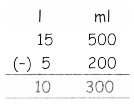Question 6.
I purchased 73 l of milk. My sister has taken 34 l 500 ml of milk. So how much milk is left for me?